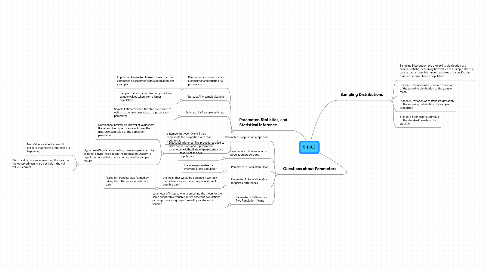9.1-9.3

Get Started. It's Free9.1-9.31. Parameter, Statistics, and Statistical Inference

1.1. Parameter: a number that is a summary characteristic of a population

1.1.1. Population Parameter: Makes it clear that the parameter is associated with a population, not a sample

1.2. Statstic: AKA sample Statistic

1.2.1. Sample Statistic: a number computed from sample values taken from a larger population

1.3. Estimate: AKA sample estimate

1.3.1. Sample Estimate: when the statistic is used to estimate the unknown value of a population parameter

1.4. Statistical Inference: The procedures used to make conclusions about population parameters on the basis of sample statistics

1.4.1. Confidence Interval: an interval of values that the researcher is pretty sure will cover the true, unknown value of the population parameter

1.4.2. Hypothesis/Significance Testing: uses sample data to try to reject a hypothesis about the population. Usually to reject the notion that chance can explain the sample results

1.4.2.1. Null Value: a value that would indicate that nothing interesting is happening

1.4.2.2. Statistical Significance: rejecting the idea that the observed results are possible ir the null value is correct

2. Questions about Parameters

2.1. Parameter 1: population proportion

2.1.1. a number between 0 and 1 that represents the proportion with that certain trait

2.2. Parameter 2: Difference in two population proportions

2.2.1. this is used to compare some feature of the two populations

2.3. Parameter 3: Population Mean

2.3.1. the average variable for everyone in the population

2.4. Parameter 4: Population Mean for paired differences

2.4.1. the mean that would be obtained if we took the differences for the entire population of possible pairs

2.4.1.1. Paired differences: data formed by taking the differences in matched pairs

2.5. Parameter 5: Difference in Two Population Means

2.5.1. parameter of interest when comparing the mean for the same quantitative variable in two different populations or two population groups formed by a categorical variable